## Sunday, November 11, 2012

### Jacobi Elliptical Functions

This blog entry is possible thanks to @mathematicsprof on Twitter. He posted an article "Jacobi Elliptic Functions from a Dynamic Systems Point of View", written by Ken Meyer Ph.D of the University of Cincinnati, which not only gave me something to read during lunch last Friday but also lead me on how to calculate Jacobi Elliptic Functions. I am so grateful!

By the way, I am on Twitter: @edward_shore.

Jacobi Elliptic Functions - An Introduction

Let k and t be parameters where 0 < k < 1 as the following system of differential equations is to be solved:

dx/dt = y(t) * z(t)
dy/dt = -z(t) * x(t)
dz/dt = -k^2 * x(t) * y(t)

which satisfy the initial conditions x(0) = 0, y(0) = 1, and z(0) = 1.

The Jacobi Elliptical Functions are defined to be the solutions to the above system.

The sine amplitude function is defined as sn(t, k) = x(t).

The cosine amplitude function is defined as cn(t, k) = y(t).

The delta amplitude function is defined as dn(t,k) = z(t).

A very interesting point, which has also managed to trip me up for years, is that there is no explicit closed formula for sn(t,k), cn(t,k), and dn(t,k). Yet mathematicians can find the derivatives and integrals of these functions.

Derivatives of the Jacobi Elliptical Functions

These derivatives, by looking at the system above, are:

d/dt sn(t,k) = cn(t,k) * dn(t,k)
d/dt cn(t,k) = -dn(t,k) * sn(t,k)
d/dt dn(t,k) = -k^2 * sn(t,k) * cn(t,k)

When k=0

By setting k=0 and finding solutions to the system equations becomes:

dz/dt = 0
z(t) = c
Since z(0)=1, conclude z(t)=1.

Then
dx/dt = y(t)
dy/dt = -x(t)
with initial conditions x(0)=0 and y(0)=1.

Since sin(0)=0, cos(0)=1, d/dt sin t = cos t, and d/dt cos t = -sin t, we can conclude that x(t) = sin t and y(t) = cos t. Meyer states that as k approaches 0, sn(t,k) approaches sin(t), cn(t,k) approaches cos(t), and dn(t,k) approaches 1.

A Very Familiar Identity

Similar to the famous identity:

sin²(θ) + cos²(θ) = 1

An identity of Jacobi Elliptical functions is:

sn²(t,k) + cn²(t,k) = 1

Why is this true? Take the derivative with respect to t and we find that:

2 sn(t,k) cn(t,k) dn(t,k) - 2 cn(t,k) dn(t,k) sn(t,k) = 0

How to Calculate

We can use the integral definition to assist us in calculating values for sn, cn, and dn. In this section, let the parameters be u and k, respectively. The integral definition stems from the incomplete elliptical integral: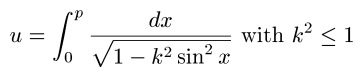where u and k are known and p is the value we are solving for.

The value p is known as the Jacobi Amplitude, am(u,k) for short. Then:

am(u,k) = p

sn(u,k) = sin(am(u,k)) = p

cn(u,k) = cos(am(u,k)) = p

dn(u,k) = √(1 - k² sin² (am(u,k)) = √(1 - k² sin²(p))

Advanced mathematics software or an online calculator, such as this one from Ke!san, are often used to calculate values of Jacobi Elliptical Functions. Fortunately, this task can be done with high-end graphing calculators, using their solve application.

I have been able to obtain accurate answers using the Hewlett Packard HP-50g, Texas Instruments TI-84+, and Casio Prizm fx-CG 10. The later two are relatively fast in finding values. Below I will show the setup for each of the three calculators. I am pretty sure the TI nSpire can handle this too. The following screens show how to obtain am(u,k), the Jacobi Elliptical functions easily follow.

In the solver screen, you can leave X blank (HP-50G only), or just assign any arbitrary value to X, such as 1, since X is the dummy variable in the integration and has no effect on calculations.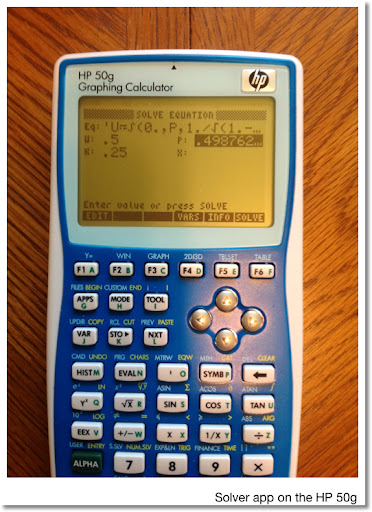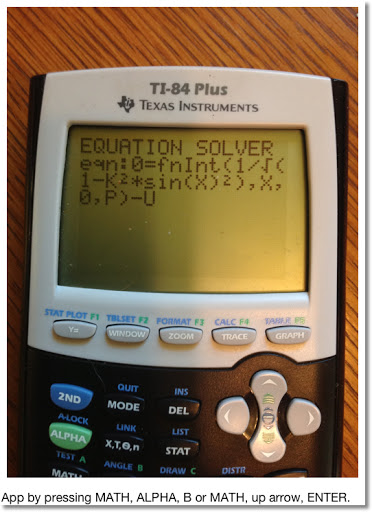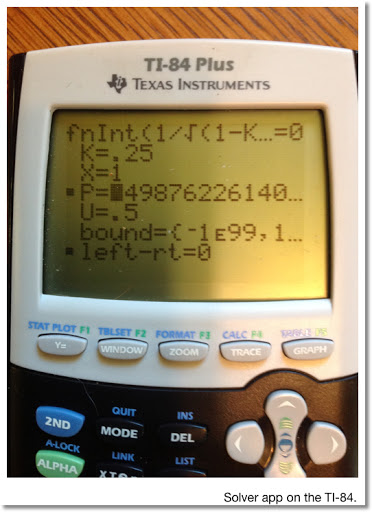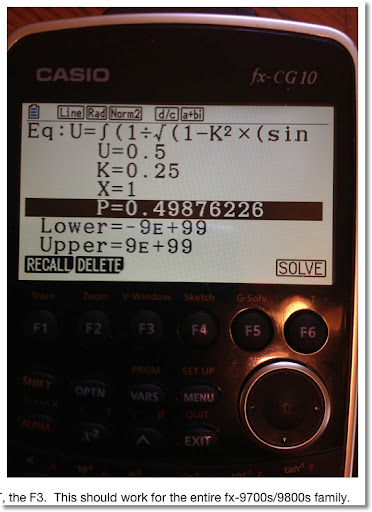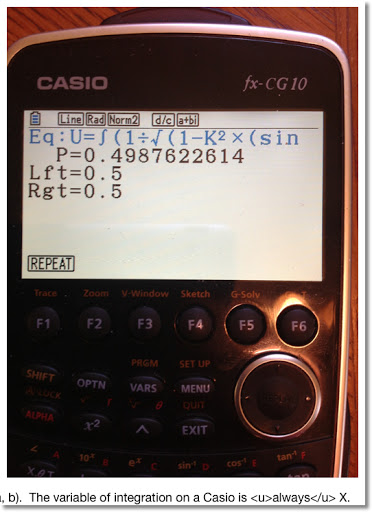Here is a small table of values: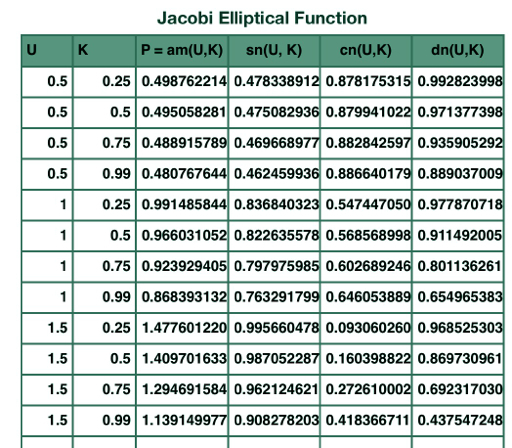Resources

Meyer, Kenneth R. "Jacobi Elliptic Functions from a Dynamic Systems Point of View" The Mathematical Association of America. Monthly 108. October 2001 - retrieved 11/9/2012

Weinstein, Eric "Jacobi Elliptic Functions" - From MathWorld - A Wolfram Web Source, http://mathworld.wolfram.com/JacobiEllipticFunctions.html, retrieved 11/11/2012

Fun as always! Thank you as always. Until next time, Eddie

This blog is property of Edward Shore, 2012.

1.2.